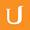## Exponential Function - Differential Equations in Action

• 0:00 - 0:09
Euler's number and the exponential function based on that number are of vital importance in the theory of differential equations.
• 0:09 - 0:16
Whenever we are dealing with exponential growth or exponential decay, and whenever we deal with oscillations,
• 0:16 - 0:22
Euler's number plays a big role. This is a brief and informed introduction to that topic, for which we need a minimum
• 0:22 - 0:29
amount of prerequisites. In particular, you should know that five to the first power equals five.
• 0:29 - 0:39
Five to the zeroth power equals one. Five to the minus second power equals one over 25.
• 0:39 - 0:44
Five to the power of one half equals the square root of five.
• 0:44 - 0:54
Five to the third power times five to the fourth power equals five to the power of three plus four--seven.
• 0:54 - 1:02
And five to the third power to the fourth power equals five to the power of 12--three times four.
• 1:02 - 1:05
This is what you need to know about powers of numbers.
• 1:05 - 1:13
In addition, you should have an idea about derivatives in this D, DX notation--for instance, the derivate of X squared
• 1:13 - 1:20
with respect to X equals 2X. And you should have an idea of how this is connected with the slope of the tangent line.
Title:
Exponential Function - Differential Equations in Action
Video Language:
English
Team:Udacity
Project:
CS222 - Differential Equations
Duration:
01:21Udacity Robot edited English subtitles for Exponential Function - Differential Equations in Actionsp1 added a translation

# English subtitles

## Revisions Compare revisions

• API
Udacity Robot
• sp1